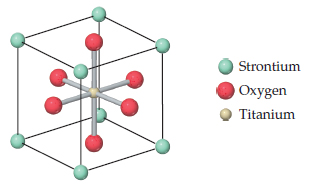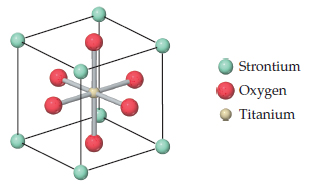# Problem: Tausonite, a mineral composed o f Sr, O, and Ti, has the cubic unit cell shown in the drawing .What is the empirical formula of this mineral?

###### FREE Expert Solution

We are asked to determine the empirical formula of Tausonite using the unit cell diagram given.A unit cell is the smallest repeating unit in a crystal lattice. Whole crystal lattice can be built by repeating the unit cell in three dimensions.

88% (184 ratings)###### Problem Details

Tausonite, a mineral composed o f Sr, O, and Ti, has the cubic unit cell shown in the drawing.

What is the empirical formula of this mineral?